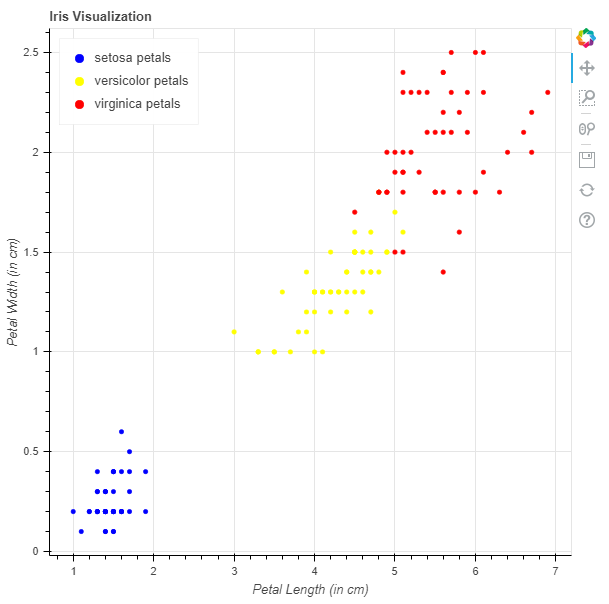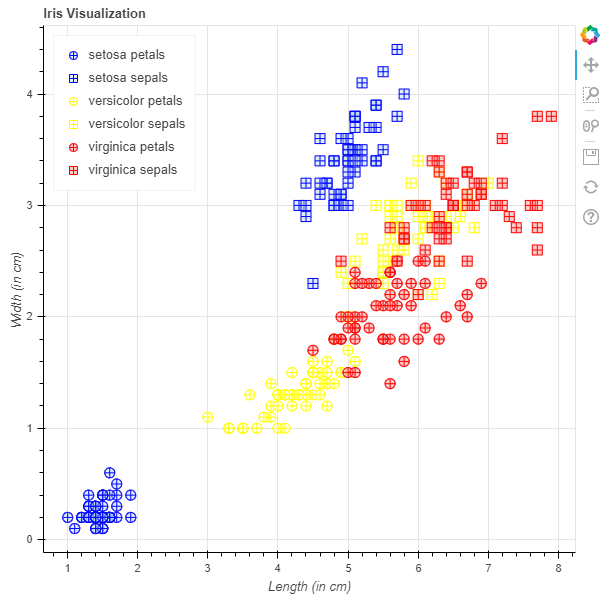Open in App
Not now

# Python Bokeh – Visualizing the Iris Dataset

• Last Updated : 29 Nov, 2022

Bokeh is a Python interactive data visualization. It renders its plots using HTML and JavaScript. It targets modern web browsers for presentation providing elegant, concise construction of novel graphics with high-performance interactivity. Bokeh can be used to visualize the Iris flower dataset. Visualization is done using the plotting module. Here we will be using the Iris dataset given to us by Bokeh.

To download the Iris dataset run the following command on the command line :

`bokeh sampledata`

Alternatively, we can also execute the following Python code :

```import bokeh

### Analyzing the dataset:

In the sample data provided by Bokeh, there is a file iris.csv, this is the Iris dataset. Below is a glimpse into the iris.csv file :

```sepal_length    sepal_width    petal_length    petal_width    species
5.1        3.5        1.4        0.2        setosa
4.9        3        1.4        0.2        setosa
4.7        3.2        1.3        0.2        setosa
4.6        3.1        1.5        0.2        setosa
5        3.6        1.4        0.2        setosa```

The dataset contains 5 attributes which are :

• sepal_length in cm
• sepal_width in cm
• petal_length in cm
• petal_width in cm
• species has 3 types of flower species :
• setosa
• versicolor
• virginica

Each species has 50 records and the total entries are 150.

### Visualizing the Dataset:

We will be plotting graphs to visualize the clustering of the data for all the 3 species.

Example 1: Here will be plotting a graph with length of petals as the x-axis and the breadth of petals as the y-axis.

1. Import the required modules:
• figure, output_file and show from bokeh.plotting
• flowers from bokeh.sampledata.iris
2. Instantiate a figure object with the title.
3. Give the names to x-axis and y-axis.
4. Plot the graphs for all the 3 species.
5. Display the model.

Example:

## Python3

 `# importing the modules``from` `bokeh.sampledata.iris ``import` `flowers``from` `bokeh.plotting ``import` `figure, show, output_file` `# file to save the model``output_file(``"gfg.html"``)` `# instantiating the figure object``graph ``=` `figure(title``=``"Iris Visualization"``)` `# labeling the x-axis and the y-axis``graph.xaxis.axis_label ``=` `"Petal Length (in cm)"``graph.yaxis.axis_label ``=` `"Petal Width (in cm)"` `# plotting for setosa petals``x ``=` `flowers[flowers[``"species"``] ``=``=` `"setosa"``][``"petal_length"``]``y ``=` `flowers[flowers[``"species"``] ``=``=` `"setosa"``][``"petal_width"``]``color ``=` `"blue"``legend_label ``=` `"setosa petals"``graph.circle(x, y,``             ``color``=``color,``             ``legend_label``=``legend_label)` `# plotting for versicolor petals``x ``=` `flowers[flowers[``"species"``] ``=``=` `"versicolor"``][``"petal_length"``]``y ``=` `flowers[flowers[``"species"``] ``=``=` `"versicolor"``][``"petal_width"``]``color ``=` `"yellow"``legend_label ``=` `"versicolor petals"``graph.circle(x, y,``             ``color``=``color,``             ``legend_label``=``legend_label)` `# plotting for virginica petals``x ``=` `flowers[flowers[``"species"``] ``=``=` `"virginica"``][``"petal_length"``]``y ``=` `flowers[flowers[``"species"``] ``=``=` `"virginica"``][``"petal_width"``]``color ``=` `"red"``legend_label ``=` `"virginica petals"``graph.circle(x, y,``             ``color``=``color,``             ``legend_label``=``legend_label)` `# relocating the legend table to``# avoid abstruction of the graph``graph.legend.location ``=` `"top_left"` `# displaying the model``show(graph)`

Output:Code Explanation:

1. The code starts by importing the modules needed for this project.
2. Next, a file is created to save the model and then an output_file() function is called with the name of that file.
3. Next, a figure object is instantiated and labeled with x-axis and y-axis labels.
4. The next step in creating this graph is plotting for setosa petals using flowers[flowers[“species”] == “setosa”][“petal_length”] .
5. Then, color = “blue”, legend_label = “setosa petals”.
6. Next, plotting for versicolor petals using flowers[flowers[“species”] == “versicolor”][“petal_length”], color = “yellow”, legend_label = “versicolor petals”.
7. Lastly, plotting for virginica petals using flowers[flowers[“species”] == “virginica”][“petal_length”], color = red,”legend label=”virginica petals.”
8. The code is a small snippet of code that will create an interactive graph of the iris flower.
9. The graph will be created using bokeh, which is a Python library for creating interactive visualizations.
10. The code starts by importing the necessary modules to run the program and then instantiating a figure object with the title “Iris Visualization”.
11. Next, labeling the x-axis and y-axis on the graph, plotting for setosa petals, versicolor petals, virginica petals and relocating the legend table to avoid abstruction of the graph.
12. Finally, displaying the model.

### Example 2:

Here will be plotting a scatter plot graph with both sepals and petals with length as the x-axis and breadth as the y-axis.

1. Import the required modules :
• figure, output_file and show from bokeh.plotting
• flowers from bokeh.sampledata.iris
2. Instantiate a figure object with the title.
3. Give the names to x-axis and y-axis.
4. Plot the graphs for all the 3 species.
5. Display the model.

## Python3

 `# importing the modules``from` `bokeh.sampledata.iris ``import` `flowers``from` `bokeh.plotting ``import` `figure, show, output_file` `# file to save the model``output_file(``"gfg.html"``)` `# instantiating the figure object``graph ``=` `figure(title``=``"Iris Visualization"``)` `# labeling the x-axis and the y-axis``graph.xaxis.axis_label ``=` `"Length (in cm)"``graph.yaxis.axis_label ``=` `"Width (in cm)"` `# plotting for setosa petals``x ``=` `flowers[flowers[``"species"``] ``=``=` `"setosa"``][``"petal_length"``]``y ``=` `flowers[flowers[``"species"``] ``=``=` `"setosa"``][``"petal_width"``]``marker ``=` `"circle_cross"``line_color ``=` `"blue"``fill_color ``=` `"lightblue"``fill_alpha ``=` `0.4``size ``=` `10``legend_label ``=` `"setosa petals"``graph.scatter(x, y,``              ``marker``=``marker,``              ``line_color``=``line_color,``              ``fill_color``=``fill_color,``              ``fill_alpha``=``fill_alpha,``              ``size``=``size,``              ``legend_label``=``legend_label)` `# plotting for setosa sepals``x ``=` `flowers[flowers[``"species"``] ``=``=` `"setosa"``][``"sepal_length"``]``y ``=` `flowers[flowers[``"species"``] ``=``=` `"setosa"``][``"sepal_width"``]``marker ``=` `"square_cross"``line_color ``=` `"blue"``fill_color ``=` `"lightblue"``fill_alpha ``=` `0.4``size ``=` `10``legend_label ``=` `"setosa sepals"``graph.scatter(x, y,``              ``marker``=``marker,``              ``line_color``=``line_color,``              ``fill_color``=``fill_color,``              ``fill_alpha``=``fill_alpha,``              ``size``=``size,``              ``legend_label``=``legend_label)` `# plotting for versicolor petals``x ``=` `flowers[flowers[``"species"``] ``=``=` `"versicolor"``][``"petal_length"``]``y ``=` `flowers[flowers[``"species"``] ``=``=` `"versicolor"``][``"petal_width"``]``marker ``=` `"circle_cross"``line_color ``=` `"yellow"``fill_color ``=` `"lightyellow"``fill_alpha ``=` `0.4``size ``=` `10``legend_label ``=` `"versicolor petals"``graph.scatter(x, y,``              ``marker``=``marker,``              ``line_color``=``line_color,``              ``fill_color``=``fill_color,``              ``fill_alpha``=``fill_alpha,``              ``size``=``size,``              ``legend_label``=``legend_label)` `# plotting for versicolor sepals``x ``=` `flowers[flowers[``"species"``] ``=``=` `"versicolor"``][``"sepal_length"``]``y ``=` `flowers[flowers[``"species"``] ``=``=` `"versicolor"``][``"sepal_width"``]``marker ``=` `"square_cross"``line_color ``=` `"yellow"``fill_color ``=` `"lightyellow"``fill_alpha ``=` `0.4``size ``=` `10``legend_label ``=` `"versicolor sepals"``graph.scatter(x, y,``              ``marker``=``marker,``              ``line_color``=``line_color,``              ``fill_color``=``fill_color,``              ``fill_alpha``=``fill_alpha,``              ``size``=``size,``              ``legend_label``=``legend_label)` `# plotting for virginica petals``x ``=` `flowers[flowers[``"species"``] ``=``=` `"virginica"``][``"petal_length"``]``y ``=` `flowers[flowers[``"species"``] ``=``=` `"virginica"``][``"petal_width"``]``marker ``=` `"circle_cross"``line_color ``=` `"red"``fill_color ``=` `"lightcoral"``fill_alpha ``=` `0.4``size ``=` `10``legend_label ``=` `"virginica petals"``graph.scatter(x, y,``              ``marker``=``marker,``              ``line_color``=``line_color,``              ``fill_color``=``fill_color,``              ``fill_alpha``=``fill_alpha,``              ``size``=``size,``              ``legend_label``=``legend_label)` `# plotting for virginica sepals``x ``=` `flowers[flowers[``"species"``] ``=``=` `"virginica"``][``"sepal_length"``]``y ``=` `flowers[flowers[``"species"``] ``=``=` `"virginica"``][``"sepal_width"``]``marker ``=` `"square_cross"``line_color ``=` `"red"``fill_color ``=` `"lightcoral"``fill_alpha ``=` `0.4``size ``=` `10``legend_label ``=` `"virginica sepals"``graph.scatter(x, y,``              ``marker``=``marker,``              ``line_color``=``line_color,``              ``fill_color``=``fill_color,``              ``fill_alpha``=``fill_alpha,``              ``size``=``size,``              ``legend_label``=``legend_label)` `# relocating the legend table to``# avoid abstruction of the graph``graph.legend.location ``=` `"top_left"` `# displaying the model``show(graph)`

Output:Code Explanation:

1. The code starts by importing the modules that are needed to run this program.
2. The first module is from bokeh.sampledata and it imports flowers, which will be used later in the code.
3. The second module is from bokeh.plotting and it imports figure, show, output_file, which are all used throughout the code to create a graph of an iris flower dataset.
4. The next line creates a file called gfg.html that will be saved after running this program so that you can view your final product on another computer or device with internet access (e.g., laptop).
5. This line also instantiates a figure object named “graph” with title “Iris Visualization”.
6. Next comes labeling the x-axis and y-axis for easy reference later on in the code as well as creating two variables: length and width for use in plotting data points onto these axes respectively; they both have axis labels set to “Length (in cm)” and “Width (in cm)”.
7. The code is used to create a graph of the Iris flower.
8. The code starts by creating a scatter graph with the x-axis and y-axis.
9. The x-axis is labeled “species” and the y-axis is labeled “petal_length.”
10. Next, it creates a marker called “circle_cross” that has a line color of blue and fill color of lightblue.
11. It also sets the size to 10.
12. Finally, it creates two legends: one for setosa petals and one for versicolor sepals.
13. The code is used to plot the setosa petals and sepals of a flower, as well as the versicolor petals and sepals.
14. The code below is used to plot the data points for both sets of petals and sepals.
15. # plotting for setosa petals x = flowers[flowers[“species”] == “setosa”][“petal_length”] y = flowers[flowers[“species”] == “setosa”][“petal_width”] marker = “circle_cross” line_color = “blue” fill_color = “lightblue” fill_alpha = 0.4
16. The code starts by creating a scatter graph with the species as the x-axis and petal length, width, and sepal length as the y-axis.
17. The first line creates a scatter graph for virginica flowers.
18. The second line creates a scatter graph for virginica sepals.
19. The code starts by creating an empty list called flowers that will hold all of the flowers in this dataset.
20. Then it loops through each flower in this list to create three variables: species, petal_length, and petal_width which are used to plot on the scatter graph later on.
21. Next it sets up two lists: one is called markers that contains different types of shapes (circle_cross, square_cross) while another is called lines that contains colors (red).
22. These two lists are then passed into Graphviz’s scatters function where they’re plotted onto their respective graphs using different parameters such as marker = “circle_cross”, line_color = “red”, fill_color = “lightcoral”, size = 10, legend label=”virginia”
23. The code is used to plot the species of flowers in a scatterplot.

My Personal Notes arrow_drop_up